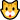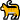# Random post:I'm trying out all the type faces.;D

177 views
:)   :D   :S. ;).    :-)      :/.  :P.  : B
commented Feb 12, 2020 by SushiMama
U forgot :3, :^, and :( .

answered Dec 29, 2019 by (709,680 points)answered Dec 28, 2019 by (6,000 points)
You forgot this one ( ͡° ͜ʖ ͡°)
answered Nov 28, 2019 by anonymous
Lol cool!
answered Jul 5, 2017 by (425,040 points)
 Haha randomized  (◕‿◕✿) (◠‿◠✿) (◠﹏◠✿) （＊＾Ｕ＾）人（≧Ｖ≦＊）/ ôヮô ∧( ‘Θ' )∧ (¤﹏¤) ●‿● ʕ·ᴥ·ʔ ＼（＾○＾）人（＾○＾）／ ヾ(＠⌒▽⌒＠)ﾉ (°∀°) ヾ｜￣ー￣｜ﾉ (☉‿☉✿) ┏(＾0＾)┛┗(＾0＾) ┓ (◡‿◡✿) ✿◕ ‿ ◕✿ ヽ(‘ ∇‘ )ノ ☆(❁‿❁)☆ ❀◕ ‿ ◕❀ ヽ(^◇^*)/ (•⊙ω⊙•) !⑈ˆ~ˆ!⑈ (*^ -^*) (⊙‿⊙✿) ◕3◕ (ﾟヮﾟ) ¢‿¢ ヅ ●ᴥ● (∪ ◡ ∪) ≖‿≖ ≧◡≦ ٩◔‿◔۶ ｡◕ ‿ ◕｡ ヾ(＠＾▽＾＠)ﾉ ◃┆◉◡◉┆▷ (✿◠‿◠) (￣ｰ￣) ╰(◡‿◡✿╰) ~,~ (ﾉ◕ヮ◕)ﾉ*:･ﾟ✧ (*~▽~) ❀‿❀ ◕‿◕ (^L^) (^▽^) ◕ ◡ ◕ (◕‿◕✿) （ ；´Д｀） ⊙﹏⊙ ✿｡✿ ヽ(゜∇゜)ノ ｡(✿‿✿)｡ (´ー｀) ツ q(❂‿❂)p ( ́ ◕◞ε◟◕`) ☆(◒‿◒)☆ (∩▂∩) (¬‿¬) (^Ｏ^) ʘ‿ʘ （'◎'） (◜௰◝) (^ｰ^) (o´ω｀o) (^з^)-☆ (◕ω◕✿) (づ｡◕‿‿◕｡)づ (ﾟ▽^*) (⌒o⌒) (｡◕‿◕｡) ت (. ﾟーﾟ) १˚◡˚५ ＼(●~▽~●) (*˘︶˘*) (✪㉨✪) (ᅌᴗᅌ* ) ^L^ (\/) (°„°) (\/) ＼(*^▽^*)/ (◠△◠✿) ( ಠ◡ಠ ) (〃^∇^)ﾉ ^^ |◔◡◉| (●⌒∇⌒●) ⊂◉‿◉つ.ʕʘ‿ʘʔ.(*・∀・*)人(*・∀・*) ＼(^-^)／ ∩(︶▽︶)∩ （☉∀☉） (´ω｀) ●﹏● （　´∀｀）☆ •ᴥ• ✿◕ ‿ ◕✿ (≧◡≦) (◡‿◡✿) (･ｪ-) ^‿^ ٩(̾●̮̮̃̾•̃̾)۶ ≖‿≖ (⊙ω⊙✿) ٩(-̮̮̃•̃) (´･ω･`) ◤(¬‿¬)◥ ^.^ (•‿•) （＾⊆＾） ^( '‿' )^ ☆d(o⌒∇⌒o)b ∑(゜Д゜;) (▰˘◡˘▰) (• ε •) ( ͡° ͜ʖ ͡°) (\/) (°,,°) (\/) (￣(エ)￣) {◕ ◡ ◕} (>‘o')> シ (❀‿❀) < (^^,) > ヾ(●⌒∇⌒●)ﾉ （　´∀｀） ☾˙❀‿❀˙☽ °٢° ^o^ (=ﾟωﾟ)ﾉ ٩(●̮̮̃•̃)۶ (☞ﾟ∀ﾟ)☞ (=゜ω゜) (｡✿‿✿｡) ó‿ó ◎[‿]◎ (▰˘◡˘▰) (︶ω︶) (ﾉ◕ヮ◕)ﾉ*:･ﾟ✧ (◠ω◠✿) ٩(^‿^)۶ (●*∩_∩*●) ٩(-̮̮̃-̃)۶ <丶´Д｀> (✿◠‿◠) ヽ(´▽｀)ノ (°⌣°) ☆(❁‿❁)☆ (० ्०) ٩(-̮̮̃•̃)۶ （╹ェ╹） ᵔᴥᵔ •(_)• ヽ(　´　∇　｀　)ノ （ミ￣ー￣ミ） (─‿‿─) ~(^з^)- (*≗*) ⊙ω⊙ (´･ω･`) (｡◕‿◕｡) .=^.^= (◠︿◠✿) ッ (`･ω･´) ´ ▽ ` )ﾉ (´∀｀) (◑‿◐) ヽ(ﾟｰﾟ*ヽ)ヽ( ˚ᆺ˚ ヽ(〃＾▽＾〃)ﾉ ｡◕‿◕｡ ❀◕ ‿ ◕❀ ( °٢° ) Ü (●´ω｀●) <('o'<) ◕‿◕
commented Nov 28, 2019 by (122,620 points)
haha I have the Japanese keyboard on my phone and I get those from there XD
answered Jul 5, 2017 by (163,520 points)
;) :) :D :P :( :-O <3 :/ ^_~ ^_^ ^0^ :-P U_U >"< O.O O.O ^o^
commented Jul 5, 2017 by (85,770 points)
Whoa , you're a type face master!
commented Feb 12, 2020 by SushiMama
i didnt relize there was so much type faces